Multiphysics CyclopediaHeat Transfer: Conservation of Energy

Chemical   Fluid Flow, Heat Transfer, and Mass Transport   Heat Transfer: Conservation Of Energy

The Energy Equation

The first law of thermodynamics defines the internal energy by stating that the change in internal energy for a closed system, ΔU, is equal to the heat supplied to the system,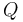, minus the work done by the system,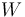:

(1)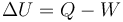If the system is allowed to move, Equation (1) can be extended to include the kinetic energy for the system,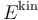:

(2)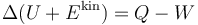Equation (2) can be written for an infinitely small volume of fluid and the result is known as the equation for conservation of total internal energy (Ref. 1):

(3)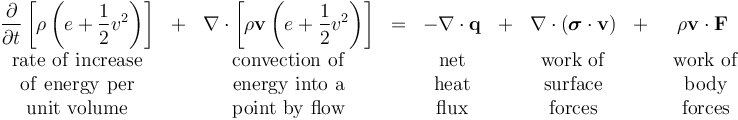In this equation:

•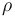is the density
•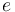is the internal energy per unit mass
•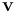is the velocity vector
•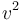is the square of the velocity magnitude
•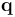is the conductive heat flux vector
•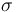is the total stress tensor
•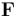is the body force per unit mass; i.e., the volume force

The total stress tensor is commonly written as:

(4)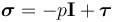where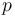denotes pressure and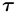is the viscous stress tensor.

Using this definition of, the second term on the right-hand side of Equation (3), which represents the work of surface forces, can be written as

(5)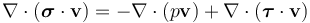The first term on the right-hand side is commonly referred to as pressure work, while the second term is known as viscous work. These terms can be further decomposed in the following way:

(6)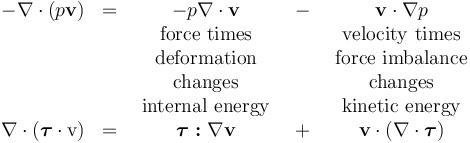The upper row in Equation (6) represents reversible effects; i.e., they can describe work that increases the internal energy and how internal energy can produce work. The bottom row describes irreversible effects; i.e., how work increases the internal energy through so-called viscous dissipation and how viscous effects reduce the kinetic energy.

Equation (3) contains the equation for conservation of kinetic energy. This equation can be derived by taking the dot product of the velocity,, with the momentum equation. Some algebraic manipulation yields: (7)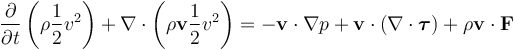It can be seen that all of the work of the body forces in the total energy equation (3) goes to change the kinetic energy. The rest of the right-hand side of Equation (7) consists of the parts of the surface force work term described in Equation (6) as affecting the kinetic energy. Subtracting Equation (7) from Equation (3) gives the equation for internal energy: (8)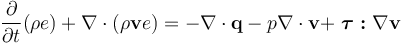In case there are internal heat sources caused by, for example, reactions or interaction with radiation, an additional internal heat source term,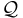, is added and the internal energy equation becomes:

(9)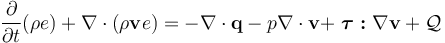The Enthalpy Equation

Internal energy is a thermodynamic state variable that is seldom used in practical applications. A more commonly used quantity is the enthalpy,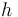, that is related to the internal energy through:

(10)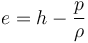Using Equation (10) in (9) and rearranging it gives the enthalpy equation (Ref. 2):

(11)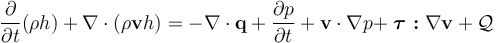Equation (11) is written in its so-called conservative form. This refers to the way that the left-hand side is written with the density and velocity inside the divergence operator. A so-called nonconservative form of the enthalpy equation can be derived by use of the continuity equation. The left-hand side of Equation (11) can be expanded in the following way:

(12)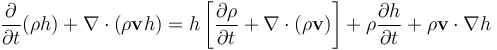The first term on the right-hand side of Equation (12) is the continuity equation multiplied by the enthalpy, and it is therefore identically zero. Equation (11) can hence be written as:

(13)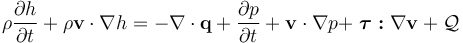Equation (13) still describes the conservation of enthalpy, even if the equation form is denoted nonconservative.

The Temperature Equation

All engineers are familiar with the concept of temperature, therefore it is convenient to express the conservation of energy in terms of temperature. Enthalpy is related to temperature,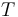, and pressure through the following differential relation:

(14)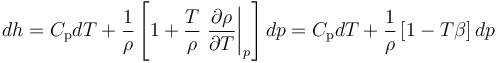where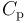is the heat capacity at constant pressure and β is the bulk expansion coefficient.

Equation (14) can be used to replacein Equation (13). Invoking the continuity equation once again gives the temperature equation:

(15)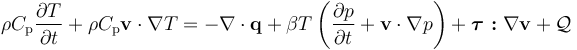The final step is to define the conduction heat flux vector,, using Fourier's law of conduction,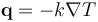, where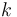is the thermal conductivity. The temperature equation then reads:

(16)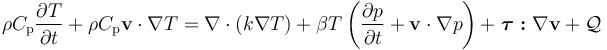Observe that the temperature equation cannot be recast on a conservative form without reverting to enthalpy or internal energy.

The temperature equation is yet another way to express conservation of energy that is mathematically equivalent to Equation (3). However, the different conservation equations do not behave equivalently when implemented using a numerical method. Many commercial codes are based on a finite volume method and they solve a transport equation on conservative form for the total enthalpy. In this way, the commercial codes achieve conservation of total energy. The total enthalpy equation, however, is prone to numerical oscillations that reduce the numerical accuracy. It is much more robust and accurate to solve the temperature equation. The finite element method allows for the solution of the temperature equation and still conserves total energy (Ref. 3).

Special Cases of Energy Conservation

For ideal gases, the term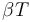is equal to unity, and (16) becomes: (17)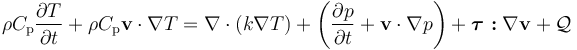If the fluid is incompressible, the pressure work term vanishes and Equation (16) reduces to:

(18)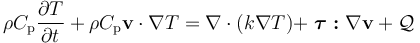The pressure work term can also be neglected for most engineering applications, unless the system undergoes a significant pressure change or if the Mach number is close to unity or higher.

The viscous heating is important in some special cases involving very high shear rates. Some engineering examples are bearings and hydraulic systems. However, viscous heating can be neglected in most other cases, and Equation (18) then simplifies further to:

(19)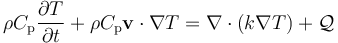Published: June 29, 2018